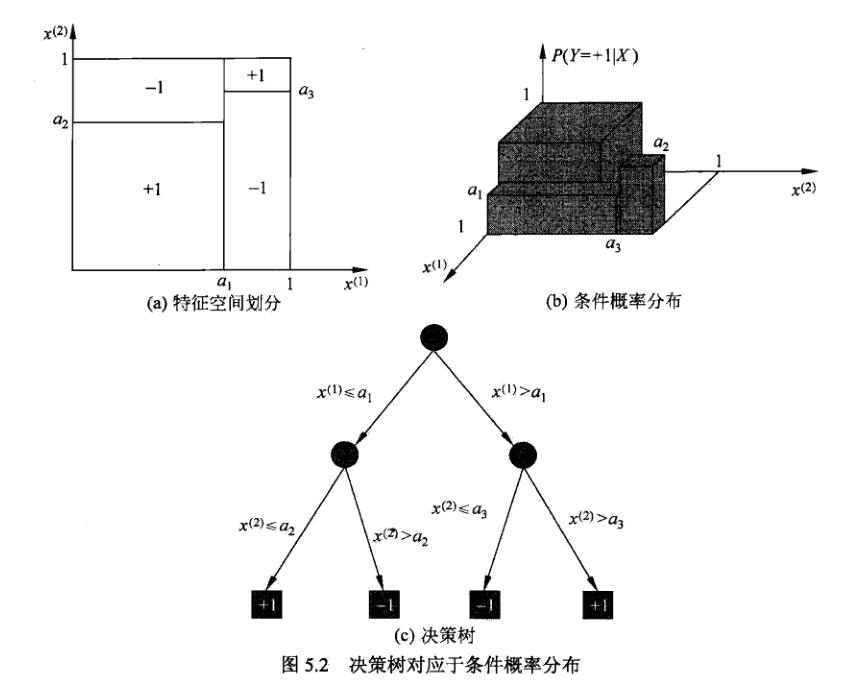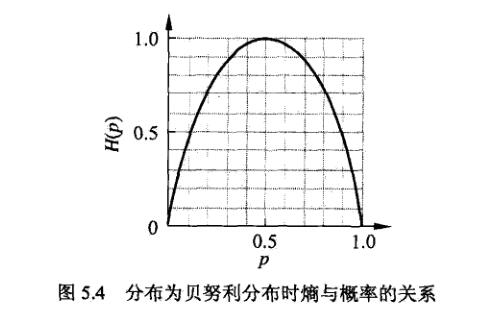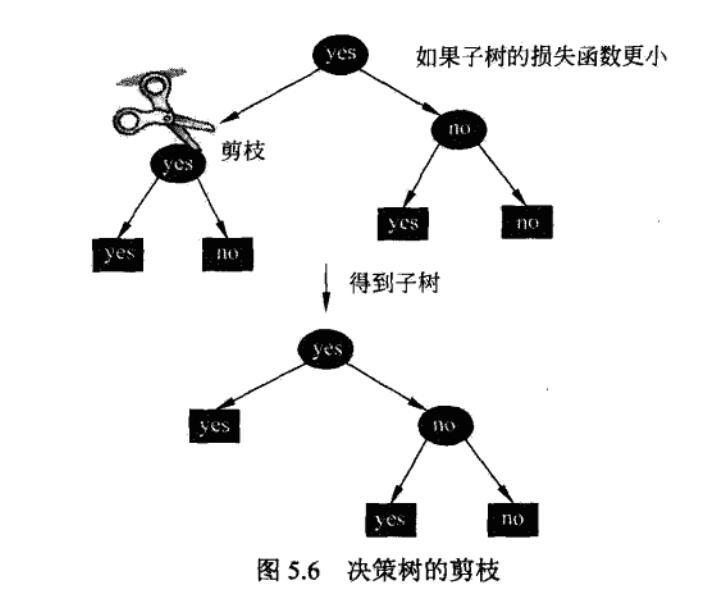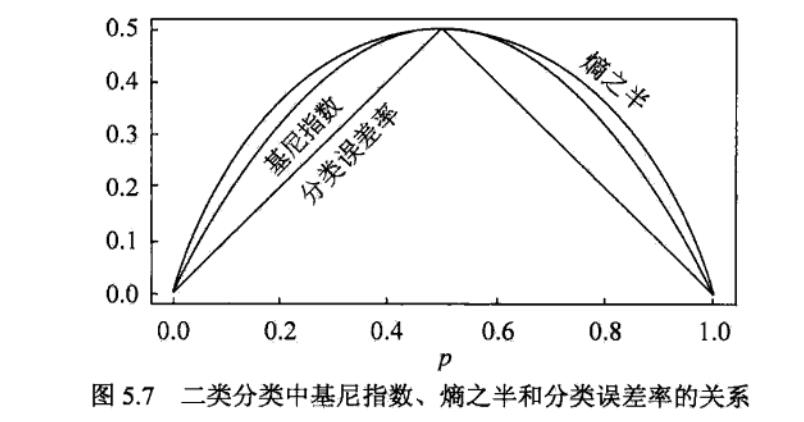# 李航-统计学习方法-笔记-5：决策树

## 基本模型

If-then：决策树路径或其对应的if-then规则集合具有一个重要的性质，互斥并且完备，也就是说，每一个实例都被一条路径或一条规则所覆盖，而且只被一条路径或者一条规则覆盖。## 特征选择

$H(x) = - \sum_{i=1}^{n} P_i \log P_i$

$$p_i=0$$时，$$0 \log0 = 0$$

$0 \leqslant H(p) \leqslant \log n$$$p=0$$$$p=1$$时，$$H(p)=0$$，随机变量完全没有不确定性。
$$p=0.5$$时，$$H(p)=1$$，熵最大，不确定性最大。

$H(Y | X) = \sum_{i=1}^{n} p_i H(Y | X = x_i)$

$g(D, A) = H(D) - H(D | A)$

$H(D) = - \sum_{k=1}^{K} \frac{|C_k|}{|D|} \log_2 {\frac{|C_k|}{|D|}}$

$H(D \ | \ A) = \sum_{i=1}^{n} \frac{|D_i|}{|D|} H(D_i) = - \sum_{i=1}^{n} \frac{|D_i|}{|D|} \sum_{k=1}^{K} \frac{|D_{ik}|}{|D_i|} \log_2 {\frac{|D_{ik}|}{|D_i|}}$

$g_R(D, A) = \frac{g(D, A)}{H_A(D)}$

$H_A(D) = - \sum_{i=1}^{n} \frac{|D_i|}{|D|} \log_2 \frac{|D_i|}{|D|}$

$$H_A(D)$$为训练集D关于特征A的值的熵，$$n$$是特征$$A$$的取值个数。

## ID3和C4.5

ID3：ID3的核心是在各个结点上应用“信息增益”准则选择特征。

C4.5：对ID3算法进行了改进，生成过程中用信息增益比来选择特征。

## 决策树的剪枝

$C_\alpha(T) = C(T) + \alpha|T|$

$\begin{split}C(T) = \sum_{t=1}^{|T|} N_t H_t(T) &= \sum_{t=1}^{|T|} N_t [- \sum_{k=1}^{K} \frac{N_{tk}}{N_t} \log \frac{N_{tk}}{N_t}] \\ &= - \sum_{t=1}^{|T|} \sum_{k=1}^{K} N_{tk} \log \frac{N_{tk}}{N_t}\end{split}$

“决策树生成”只考虑了通过提高信息增益（或信息增益比）对训练数据进行更好的拟合。而“决策树剪枝”通过优化损失函数，还考虑了减小模型复杂度。“决策树生成”学习局部的模型，而“决策树剪枝”学习整体的模型。

（1）计算每个结点的经验熵。
（2）递归地从树的叶结点向上回缩，设一组叶结点回缩到其父结点之前与之后的整体树为$$T_B$$$$T_A$$，如果

$C_\alpha(T_A) \leqslant C_\alpha(T_B) \tag{5.15}$

（3）返回（2），直至不能继续，得到损失函数最小的子树$$T_\alpha$$

$$(5.15)$$只考虑两个树的损失函数的差，其计算可以在局部进行，所以剪枝算法可由一种动态规划算法实现。## CART算法

CART：cart（classification and regression tree），分类与回归树，是应用广泛的决策树学习方法，既可用于分类，也可用于回归。

CART假设决策树是二叉树，内部结点特征的取值为“是”和“否”，左分支是取值为“是”的分支，右分支是取值为“否”的分支。这样的决策树等价于递归地二分每个特征，将输入空间即特征空间划分为有限个单元，并在这些单元上确定预测的概率分布。CART算法分为两步：

（1）决策树生成：基于训练数据集生成决策树，生成的决策树要尽量大。
（2）决策树剪枝：用验证数据集对已生成的树进行剪枝并选择最优子树，这时用损失函数最小作为剪枝的标准。

$f(x) = \sum_{m=1}^{M} c_m I(x \in R_m)$

$\hat{c}_m = ave(y_i \ | \ x_i \in R_m)$

$R_1(j, s) = \{x \ | \ x^{(j)} \leqslant s\}, \ R_2(j, s) = \{x \ | \ x^{(j)} > s\}$

$\min_{j, s} \ [ \min_{c_1} \sum_{x_i \in R_1(j,s)} (y_i - c_1)^2 + \min_{c_2} \sum_{x_i \in R_2(j,s)} (y_i - c_2)^2]$

$\hat{c}_1 = ave( y_i \ | \ x_i \in R_1(j, s)), \ \hat{c}_2 = ave( y_i \ | \ x_i \in R_2(j, s))$

$Gini(p) = \sum_{k=1}^{K}p_k (1 - p_k) = 1 - \sum_{k=1}^{K} {p_k}^2$

$Gini(p) = 2p(1-p)$

$Gini(D) = 1 - \sum_{k=1}^{K} (\frac{|C_k|}{|D|})^2$

$Gini(D, A) = \frac{|D_1|}{|D|} Gini(D_1) + \frac{|D_2|}{|D|} Gini(D_2)$

Gini值越大，样本集合的不确定性也越大（与熵类似），因此每次选择Gini小的特征来划分。## CART剪枝

（1）首先从生成算法产生的决策树$$T_0$$底端开始不断剪枝，直到$$T_0$$的根结点，形成一个子树序列$$\{T_0, T_1, ..., T_n\}$$
（2）通过交叉验证法在独立的验证数据集上对子树序列进行测试，从中选择最优子树。

$$|T|$$为子树的叶结点个数，$\alpha \geqslant 0$为参数，权衡训练数据的拟合程度与模型的复杂程度。

（1）对固定的$$\alpha$$，一定存在使损失函数$$C_\alpha(T)$$最小的子树，将其表示为$$T_\alpha$$

$$\alpha$$大时，最优子树$$T_\alpha$$偏小；$$\alpha$$小时，最优子树$$T_\alpha$$偏大。

$$\alpha = 0$$时，整体树是最优的，$$\alpha \to \infty$$时，根结点组成的单结点是最优的。

Breiman等人证明：可以用递归的方法对树进行剪枝。将$$\alpha$$从小增大，$$0 = \alpha_0 < \alpha_1 < ... < \alpha_n < + \infty$$，产生一系列的区间$$[\alpha_i, \alpha_{i+1}), i = 0, 1, ..., n$$；剪枝得到的子树序列对应着区间$$\alpha \in [\alpha_i, \alpha_{i+1})$$的最优子树序列$$\{T_0, T_1, ..., T_n\}$$

$C_\alpha(t) = C(t) + \alpha$

$$t$$为根结点的子树$$T_t$$的损失函数是：

$C_\alpha(T_t) = C(T_t) + \alpha | T_t |$

$$\alpha = 0$$及充分小时（$$\alpha < \frac{C(t) - C(T_t)}{|T_t| - 1}$$）：

$C_\alpha(T_t) < C_\alpha(t) \tag{5.29}$

$$\alpha$$增大，$$\alpha = \frac{C(t) - C(T_t)}{|T_t| - 1}$$时有：

$C_\alpha(T_t) = C_\alpha(t)$

$$\alpha$$再增大时（$$\alpha > \frac{C(t) - C(T_t)}{|T_t| - 1}$$），不等式(5.29)反向。

$g(t) = \frac{C(t) - C(T_t)}{|T_t| - 1}$

（2）利用独立的验证数据集，测试子树序列$$T_0, T_1, ..., T_n$$中各棵子树的平方误差或基尼指数。平方误差或基尼指数最小的决策树被认为是最优的决策树。子树序列$$T_1, T_2, ..., T_n$$都对应于一个参数$$\alpha_1, \alpha_2, ..., \alpha_n$$。所以，当最优子树$$T_k$$确定时，对应的$$\alpha_k$$也确定了，即得到最优决策树$$T_\alpha$$

posted @ 2019-06-05 15:33  PilgrimHui  阅读(2211)  评论(0编辑  收藏  举报